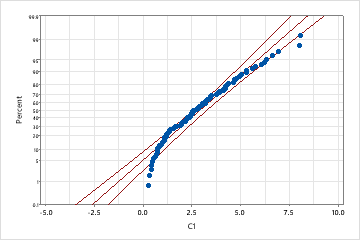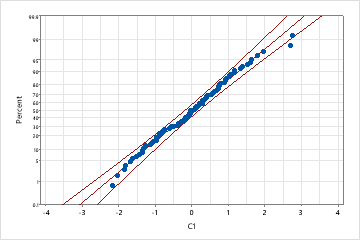## Choose between a nonnormal analysis or a normal analysis with a transformation

If you have nonnormal data, there are two approaches you can use to perform a capability analysis:
• Select a nonnormal distribution model that fits your data and then analyze the data using a capability analysis for nonnormal data, such as Nonnormal Capability Analysis.
• Transform the data so that the normal distribution is an appropriate model, and use a capability analysis for normal data, such as Normal Capability Analysis.

## Choose an appropriate distribution for your capability analysis

Selecting an appropriate distribution is an essential first step in conducting a capability analyses. If the chosen distribution does not fits the data well, then the capability estimates will be inaccurate.

• Use engineering or historical knowledge of your process.

Most often, it is best to use engineering and historical knowledge of your process to identify a distribution that fits your process data. For example, does the data follow a symmetric distribution? What distribution has worked in the past for similar situations?

• Use the Anderson-Darling test.

Sometimes it can be difficult to determine the best distribution based on the probability plot and goodness-of-fit measures. Use the Table of Percentiles from Individual Distribution Identification for several selected distributions to see how your conclusions change depending on the distribution chosen.

• Evaluate how different distributions affect your conclusions.

If several distributions provide an adequate fit to the data and similar conclusions, then it probably does not matter which distribution you choose. Conversely, if your conclusions differ depending on the distribution chosen, you may want to report the most conservative conclusion or collect more information.

## Use Individual Distribution Identification to find an appropriate distribution or transformation

Use Individual Distribution Identification prior to performing a capability analysis to determine which distribution or transformation is most appropriate for your data.

1. Choose Stat > Quality Tools > Individual Distribution Identification.
2. Choose whether your data are arranged in a single column or across rows.
3. Choose Use all distributions and transformations or Specify and choose up to 4 distributions and transformations to test.
If a nonnormal distribution provides the best fit, use one of the following nonnormal capability models to evaluate your process:
• Nonnormal Capability Analysis
• Nonnormal Capability Analysis for Multiple Variables
• Nonnormal Capability Sixpack
When you set up the analysis, indicate the type of nonnormal distribution that provided that best fit for your data.
If a transformation is most effective for your data, use one of the following normal capability models to evaluate your process:
• Normal Capability Analysis
• Normal Capability Sixpack
• Normal Capability Analysis for Multiple Variables
• Between/Within Capability Analysis
When you set up a normal capability analysis, click Transform and indicate whether to use the Johnson or the Box-Cox transformation to make your data follow a normal distribution. When you set up a between/within capability analysis, click Box-Cox and use the Box-Cox transformation to make your data follow a normal distribution

## Example of using Individual Distribution Identification to compare fits of distributions and transformations

For example, an engineer collects data on the extent of warping in ceramic tiles. The data distribution is unknown, so she performs Individual Distribution Identification on the data to compare goodness-of-fit between the exponential distribution and the normal distribution after a Johnson transformation.Exponential distribution This probability plot indicates that the exponential distribution is not a good fit; the p-value is low enough to reject the null hypothesis that the data follow an exponential distribution.Normal distribution with Johnson transformationHowever, after applying a Johnson transformation, the data closely follow a normal distribution because the p-value is large and almost all data points fall within the confidence bounds of the normal probability plot. Of these two distributions, the normal distribution with a Johnson transformation provides the better fit for your data. Thus the appropriate analysis is a normal capability analysis with a Johnson transformation.

## A comparison of normal and nonnormal capability models

Consider the following when deciding whether to use a nonnormal distribution or a normal distribution with a transformation:
• Generally, you should choose the model that is most effective for your data. If a nonnormal distribution or a transformation are equally effective, some practitioners recommend using a nonnormal model because it uses the actual data units. However, others may prefer the normal model because it provides estimates of both overall and within process capability.
• If you plan to perform repeated capability analyses on your process over time, try to choose a distribution or transformation that is likely to adequately characterize your process consistently over time. Using the same distribution or transformation lets you easily and directly compare the indices from the repeat analyses.
Normal capability
• Uses actual or transformed data for the histogram.
• Calculates within, between/within (when both within-subgroup and between-subgroup variation exists), and overall capability.
• Draws a normal curve on the histogram to help you determine whether the transformation was effective in making the data follow a normal distribution.
Nonnormal capability
• Uses actual data units for the histogram.
• Calculates only overall capability.
• Draws the chosen nonnormal distribution curve on the histogram to help you determine whether the data fits the specified distribution.
By using this site you agree to the use of cookies for analytics and personalized content.  Read our policy